Portfolio Risk and Return Part I | IFT World
IFT Notes for Level I CFA® Program
IFT Notes for Level I CFA® Program

# Part 2

## 7. Historical Return and Risk

Historical return is the return actually earned in the past, while expected return is the return one expects to earn in the future.

Historical data shows that higher returns were earned in the past by assets with higher risk. Of the three major asset classes in the U.S., namely stocks, bonds, and T-bills, it has been observed that stocks had the highest risk and return followed by bonds, and T-bills had the lowest return and lowest risk.

Asset Class

Annual Average Return Standard Deviation (Risk)
Small-cap stocks

High

Low

High

# ↓

Low

Large-cap stocks
Long-term corporate bonds
Long-term treasury bonds
Treasury bills

## 8. Other Investment Characteristics

In evaluating investments using mean and variance, we make the following two assumptions:

• Assumption 1: Distributional characteristics.
Returns are normally distributed. If this assumption does not hold, then consider skewness and kurtosis. These concepts have been covered earlier in quantitative methods.
• Assumption 2: Market characteristics.
Markets are informationally and operationally efficient. If markets are not operationally efficient, then it leads to: 1) high transaction costs 2) wide bid-ask spreads and 3) larger price impact of trades.

## 9. Risk Aversion and Portfolio Selection & The Concept of Risk Aversion

### 9.1 The Concept of Risk Aversion

Risk aversion refers to the behavior of investors preferring less risk to more risk.

Risk tolerance is the amount of risk an investor is willing to take for an investment. High risk aversion is the same as low risk tolerance. Three types of risk profiles are outlined below:

• Risk Seeking: A risk seeking investor prefers high return and high risk. Given two investments, A and B, where both have the same return but investment A has higher risk, he will prefer investment A.
• Risk Neutral: A risk neutral investor is concerned only with returns, and is indifferent about the risk involved.
• Risk Averse: A risk averse investor will prefer an investment that has lower risk, all else equal. Given two investments, A and B, where both have the same return but investment A has higher risk, he will prefer investment B.

## 10. Utility Theory and Indifference Curves

The utility of an investment can be calculated as:

Utility = E(r) – 0.5 x A x ?2

where:

A = measure of risk aversion (the marginal benefit expected by the investor in return for taking additional risk. A is higher for risk-averse individuals.

𝜎2 = variance of the investment

E(r) = expected return

Instructor’s Note:

While using this formula, use only decimal values for all parameters.

Example

An investor with A = 2 owns a risk-free asset returning 5%. What is his utility?

Solution:

Utility = 0.05 – 0.5 x 2 x 0 = 0.05

Now, he is considering an asset with 𝜎 = 10%. At what level of return will he have the same utility?

0.05 = E(r) – 0.5 x 2 x 0.12. Solving for E(R) we get 0.06 or 6.00%.

Given a choice between a risk-free asset and stock with an expected return of 10% and 𝜎 = 20%, what will he prefer?

U = 0.1 – 0.5 * 2 * 0.22 = 0.06. Since the utility number of 0.06 is higher than 0.05, the investor will prefer this investment over the previous one.

Indifference Curves

The indifference curve is a graphical representation of the utility of an investment. An indifference curve plots various combinations of risk-return pairs that an investor would accept to maintain a given level of utility. If the combinations of risk-return on a curve provide the same level of utility, then the investor would be indifferent to choosing one. Each point on an indifference curve shows that the investor is indifferent to what investment he chooses (risk/return combination) as long as the utility is the same.

To understand the indifference curve, let us plot all these numbers – expected return, standard deviation, and utility – on a graph.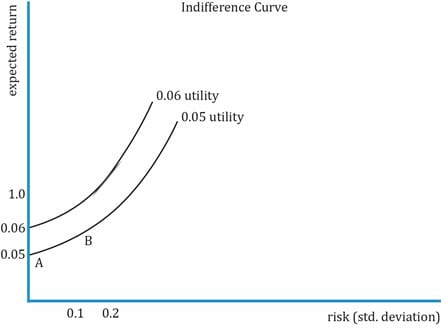The key points to note are:

• The indifference curves run from south-west to north-east. This is intuitive because for taking on higher risk (going east), investors expect to be compensated with higher expected return (going north).
• The utility of points A and B are 0.05. This means that the investor will be indifferent about choosing an investment with E(r) of 5%, ? = 0 versus another with E(r) of 6%, ? = 10% as both have the same utility. Similarly, for assuming higher levels of risk, the investor is compensated with higher return as shown in the 0.05 utility curve. Along this curve, the investor is indifferent about choosing one point (investment) over the other.
• As the investor moves north-west, he is happier as his utility increases, in this case from 0.05 to 0.06. Risk is compensated with higher returns and it signifies higher risk aversion.

We now consider the indifference curves for different types of investors.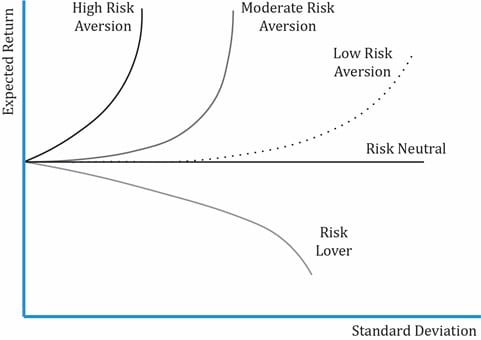The exhibit above shows the indifference curves for different types of investors with expected return on y-axis and standard deviation on x-axis. Note the following:

• Risk-neutral investor: For a risk-neutral investor, the utility is the same irrespective of risk as the investor is concerned only about the return. The expected return is constant.
• Risk-averse investor: For a risk-averse investor, the curve is upward sloping, as the investor expects additional return for taking additional risk. Finance theory assumes that most investors are risk averse, but the degree of aversion may vary. The curve is steeper for investors with high risk-aversion.
• Risk-seeking investor: The curve is downward sloping as the expected return decreases for higher levels of risk. It is not commonly seen.

## 11. Application of Utility Theory to Portfolio Selection

Now that we have seen utility theory and indifference curves for various investors, let us see how to apply it in portfolio selection. The simplest case is when a portfolio comprises two assets: a risk-free asset and a risky asset. For a high-risk averse investor, the choice is easy, to invest 100% in the risk-free asset but at the cost of lower returns. Similarly, for a risk-lover it would be to invest 100% in the risky asset. But is it the optimal allocation of assets, or can there be a trade-off?

Example

Consider a simple portfolio of a risk-free asset and a risky asset. Plot the expected return of the portfolio against the risk of the portfolio for different weights of the two assets.

 Risk-free asset Risky asset Rf = 5% Ri = 10% ? = 0 ?i = 20%

Solution:

A portfolio’s standard deviation is calculated as:

?=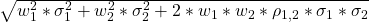Given that ?1 = 0, the terms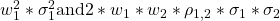become zero as the correlation between a risk-free asset with risky asset is zero.

So, the equation becomes ?p = w2 * ?2. For different weights of the risky asset, let’s calculate the values for risk and return and plot portfolio risk-return on a graph. Note that the expected return is a weighted average of the two assets.

 Weight of risky asset = w2 ?p (portfolio risk) = w2 * ?2 Rp (Portfolio return) = w1* Rf + w2 * Ri 0 0 5% 0.25 5% 6.25% 0.5 10% 7.5% 1 20% 10%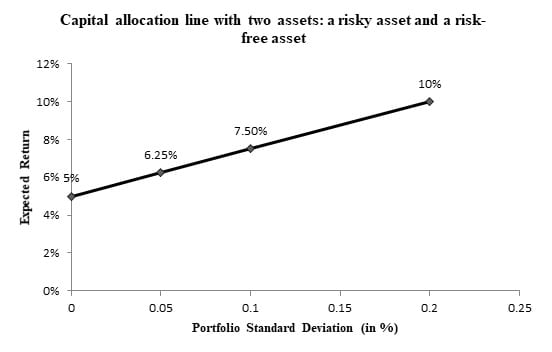Graph interpretation:

• What you get by plotting risk and return values for different weights of the risky and risk-free asset is a straight line (linear).
• As you move north-east, the weight of the risky asset increases. The lowest point on the left corner, intersecting the y-axis with expected return of 5%, represents 100% in the risk-free asset. Similarly, the topmost point on the right corner represents 100% in the risky asset with expected return of 10%.
• The straight line you see in the chart above is called the capital allocation line which represents the portfolios available to the investor as each point on the line is an investable portfolio.
• So, how does an investor decide which portfolio to invest in? The answer is by combining an investor’s indifference curves and CAL to determine the optimal portfolio. We look at it in detail in the next section.

What is the Optimal Portfolio?
We get the optimal portfolio by combining the indifference curves and the capital allocation line. The utility theory gives us the indifference curves for an individual, while the capital allocation lines give us a set of feasible investments. The optimal portfolio for an investor will lie somewhere on the capital allocation line, i.e., some combination (weight) of the risky asset and risk-free asset. Using an investor’s risk-aversion measure A, we can use the utility theory to plot the indifference curves for an individual. Assume the indifference curves for this investor look as shown in the exhibit below: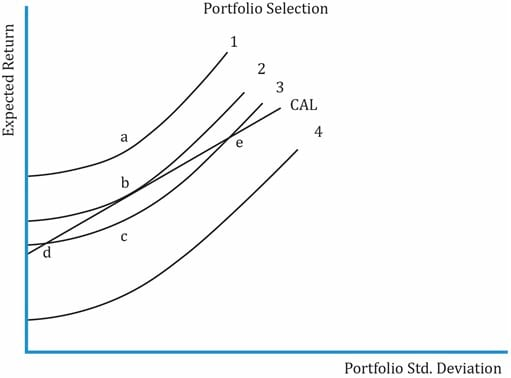Some key points about the graph:

• Curves 1, 2, 3, and 4 are various indifference curves for an individual. The indifference curves cannot intersect each other.
• Curve 1 lies above the CAL. Curve 2 is tangential to the CAL at point b. Curve 3 intersects CAL at two points: d and e. Curve 4 lies below the CAL.
• Curve 1 has the highest utility while Curve 4 has the lowest utility. As we discussed before, the investor is happier when we move north-west. Of the four curves, he will be happiest with curve 1. But, as curve 1 lies outside the CAL, it is not possible to construct a portfolio at any point on this curve with the available assets.
• Points on curve 3 may be attainable with the two assets. To achieve the utility level of curve 3, the investor may choose to invest at points d and e where it intersects with CAL.
• However, curve 2 is preferred over curve 3, as it provides a higher utility. To achieve this utility level, the investor may choose to invest at point b, where the indifference curve is tangential to the capital allocation line
• This point, b, represents the optimal portfolio.

If another investor has a higher level of risk aversion, where will his optimal portfolio lie? With a higher risk aversion level, the indifference curves will be steeper. The tangential point will be closer to the risk-free asset, so it will be to the south-west of point b.

Crash Courses for November CFA Level I, II exams are coming soon!
This is default text for notification bar